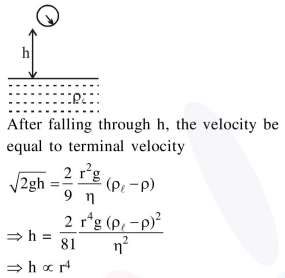# In an experiment to verify Stokes law,`
Question:

In an experiment to verify Stokes law, a small spherical ball of radius $r$ and density $\rho$ falls under gravity through a distance $h$ in air before

entering a tank of water. If the terminal velocity of the ball inside water is same as its velocity just before entering the water surface, then the value of

$\mathrm{h}$ is proportional to :

(ignore viscosity of air)

1. $\mathrm{r}$

2. $r^{4}$

3. $r^{3}$

4. $r^{2}$

Correct Option: , 2

Solution: